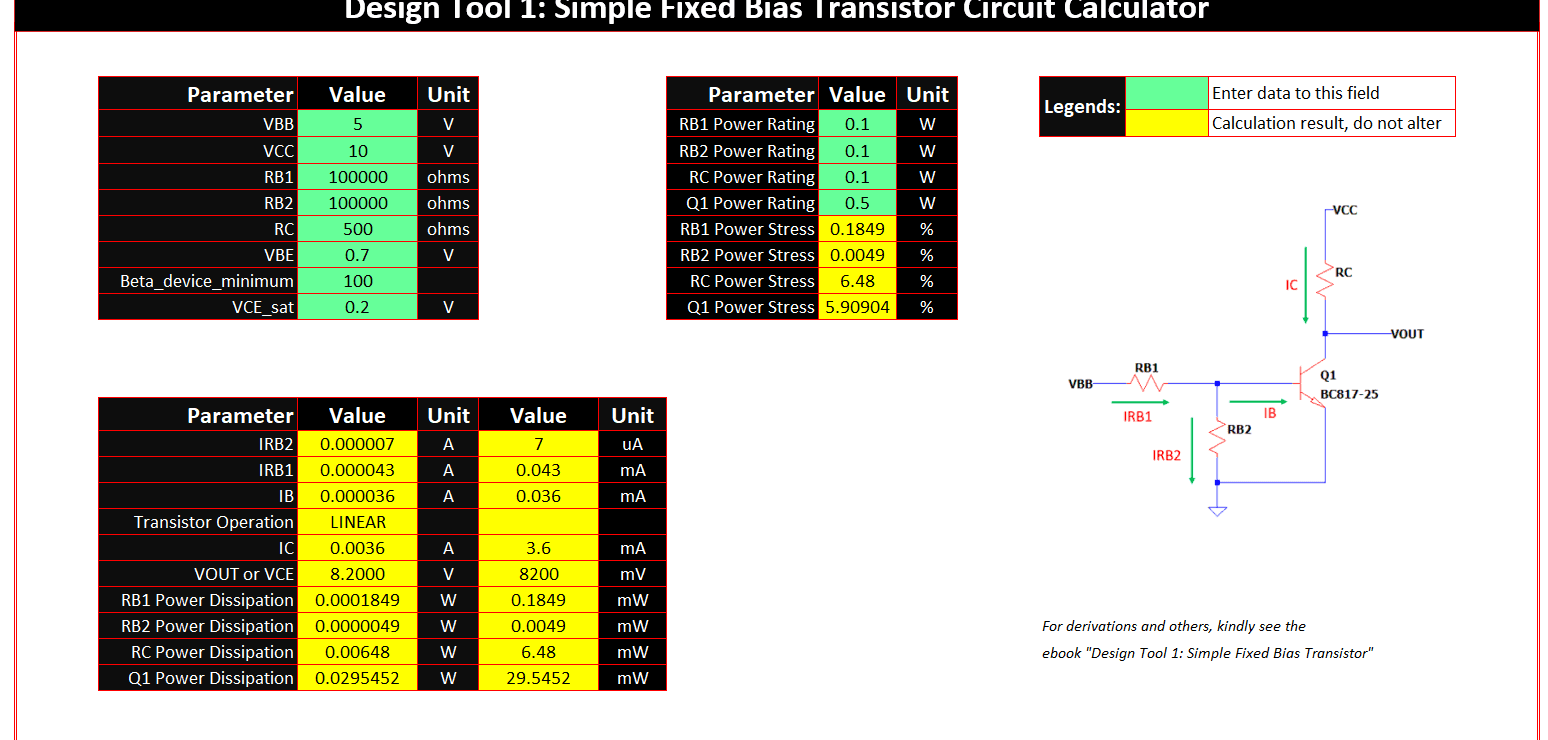# NPN Fixed Bias Design Template

Need to design a transistor fixed bias circuit using NPN? No need to go back to your university days and spend another sleepless night to derive the equations. Use the NPN Fixed Bias Design Template instead. This circuit configuration of NPN transistor is very useful in electronic switch. It has a guaranteed logic high or low when biased properly. This circuit also is can be used in linear operations or amplifier mode by changing the biasing.

This design template will automatically display the device currents; just see the reference picture to which current is to which device. The template also extracts the power dissipations and stresses as well as collector-emitter voltage.

The template has two forms. One is in MATHCAD while the other one is in EXCEL. In Mathcad, all the explanations are provided. There is also a PDF copy of the Mathcad template so that the user can still see the derivations if ever he does not have the Mathcad software.

## NPN Fixed Bias Design Template – Mathcad Derivation

The Mathcad version used in the template creation is Mathcad 14. The design template is valid only for the circuit diagram shown below. If you don’t need the RB2, you can set it to a very high value simulating an open circuit. Keep in mind not to make it zero.

### State of the Transistor – Linear or Saturation?

The design template includes how to check the transistor if it is operating in linear or saturation. You like the linear operation for amplifier while saturation for switch operation. This NPN fixed bias design template is very versatile indeed.

### Current and Power Dissipations Derivations and Stresses

All the currents present in the circuit is derived. These currents will be useful to the design like in sizing the components and setting the correct operation perhaps. Don’t waste time anymore to brainstorm how to derive these currents. They are all automatically provided in the design template.

### NPN Fixed Bias Design Template in Mathcad

This is how the NPN Fixed Bias Design Template works. Input all values to the green fields. Follow the circuit diagram for the variables. RB1_1, RB2_2 and RC_1 are just referring to RB1, RB2 and RC. Used the underscore 1 and 2 in Mathcad template since there are two examples provided and Mathcad will not allow same variable name.

All the yellow fields on the other hand are computation results. Do not alter them. All the pink fields are just denoting key equations.

The design template in Mathcad when you open it as is, there are two examples. First is to demonstrate a saturation state (for switch) and the second one is to show a linear state (for amplifier).

## NPN Fixed Bias Design Template – Excel

You have no Mathcad? No problem. You can use the excel version. It will give the same result. It is very handy for most of the user especially to those who don’t want to see anymore the equations and derivations behind. However, if you still need to see the derivations, there is a PDF copy of the Mathcad derivations provided together in the package.

## Design Sample Using the Excel Template

In this example, we will use the transistor PBSS4160T-QR from Nexperia. Assuming the ambient temperature where the design is to put is 50’C, then the resulting power dissipation of this transistor is

Power dissipation, de-rated = (Tjmax – Tamax) / Rthja = (150’C – 50’C) / 312K/W = 0.321 W

Where Tjmax and Rthja are both provided in the datasheet as below image.

We will put the 0.321 W under the field Q1 Power Rating of the NPN Fixed Bias Design Template in excel. From the datasheet also, the minimum beta is 100, and put this to the excel template under Beta_device_minimum.

For the VCEsat and VBE, I am considering for both the maximum values and put to the designated fields.

With the other inputs in green fields, the power stress on Q1 is 1.72%. As simple as that!

When you download the NPN Fixed Bias Design Template and run into troubles, please email to [email protected]. We are happy to help you. We are also open for consultations.